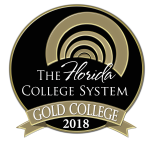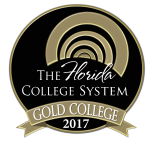MAC1311

## Calculus and Analytic Geometry I

Course Code:
MAC1311

Credit Hours:
4

Effective beginning:
2023-24

Sections:
001

Course Description:
This is a course including analytic geometry of the line and circle, limits, continuity, derivatives and integrals of the algebraic and transcendental functions, applications of integrals to finding area and volume, exponential growth and decay, Riemann sums and the Riemann integral, trapezoidal and Simpson’s Rule.

### Course Details

##### Prerequisites:

Prerequisite: A “C” or higher in MAC 1114 and MAC 1140 or consent of the department. A “C” grade or higher must be earned to advance to a higher level mathematics course or to satisfy part of the general education requirements in mathematics.

##### Instructors:

Lisa Purvee
purveel@chipola.edu

##### Required textbooks/ course materials:

Calculus, 12th Edition, by Larson, Cengage Learning Enhanced WebAssign Access Card, ISBN: 9780357749135

or

eBook, ISBN: 9780357749401

##### Assignment/course outline:

See first-day handout.

##### Discipline-level learning outcomes:

Area 3 -Math:   Demonstrate Basic Mathematical Skills and Knowledge

The purpose of the mathematics component of the core curriculum is to develop quantitative literacy with an ability to apply basic mathematical tools in the solution of real-world problems.

M-1 Solve mathematical problems by using arithmetic, algebraic, or geometric skills.

M-2 Translate basic mathematical information verbally, numerically, graphically, or symbolically.

M-3 Solve mathematical problems using appropriate technology.

M-4 Interpret mathematical models such as formulas, graphs, tables, or schematics.

M-5 Solve contextual problems using mathematical processes.

##### Course-level learning outcomes:

Course-level student learning outcomesDiscipline-level learning outcomesAssessment methods
Find limits numerically, graphically, and algebraically. M-1, M-2 Unit Tests, Cumulative Final, Homework, Dept. Exam
Calculate derivatives of functions using rules of differentiation. M-1, M-2 Unit Tests, Cumulative Final, Homework, Dept. Exam
Use properties of differentiation to identify the characteristics of functions such as extrema and/or points of inflections. M-1, M-3 Unit Tests, Cumulative Final, Homework, Dept. Exam
Calculate indefinite and definite integrals. M-1, M-2, M-3 Unit Tests, Cumulative Final, Homework, Dept. Exam
Solve applied problems using derivatives and integrals M-1, M-2, M-3, M-4, M-5 Unit Tests, Homework

##### Means of accomplishing learning outcomes:

Teacher Facilitated: The teacher will be leading class discussions on the material contained in the text during each class period.

Student-Centered: The students will be solving problems during each class period using their own graphing calculators.

Office Hours: The instructor will be available during office hours for individual assistance.  The instructor’s schedule can be found posted on their office door and/or via their individual web site.

ACE Tutors: Student tutors are available to provide individualized help.  Hours can be found posted each semester on the lab door and/or via the web site.

### College-wide policies and resources

For more specific information on Chipola's college-wide academic policies and resources available to students, visit the link below.Chipola College
3094 Indian Circle
Marianna, FL 32446
850.526.2761